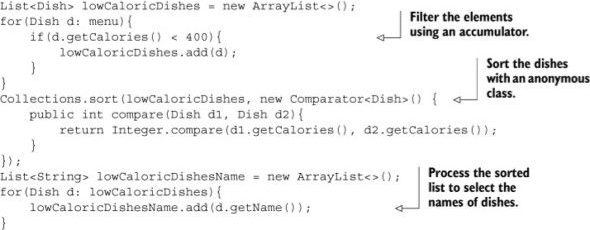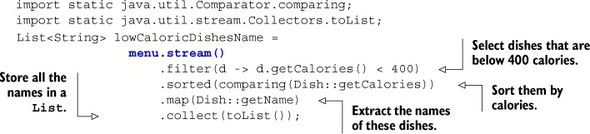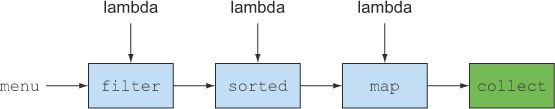Go Back

# Lambda expressions and streams

#### Posted by Tom Spencer on 2019-12-29

In this blog we look mainly at Chapter 3 of Java SE 8 for the Really Impatient by Cay S. Horstmann. This chapter is about how to write lamda expressions correctly and what you can and can’t do with these expressions.

#### Definition of lambda expression:

A lambda expression can be understood as a concise representation of an anonymous function that can be passed around: it doesn’t have a name, but it has a list of parameters, a body, a return type, and also possibly a list of exceptions that can be thrown.

Lambda expressions can be passed around and have a specific syntax:

``````(Apple a1, Apple a2) -> a1.getWeight().compareTo(a2.getWeight());
``````

The body of a lambda expression is usually curly brackets but if it has one line just writing the line is enough.

#### 3.2 Where and how to use lambdas

We use lambdas to send functions as parameters to other functions. In order to do that Java designed the concept of a functional interface. An interface is a class which is abstract ie none of its functions are defined ie the names are defined not the functionality of the names. A functional interface is an interface which has defined abstract methods. The rule for a functional interface here is that it has to have one abstract method only and the defined methods have to be labelled default.

#### Polymorphism with functional interfaces

Polymorphism is when classes have different methods but same name taking different types. In this example we see a practical example of polymorhpism in Ruby. Our user is going to select an option from a list of possible actions relating to a Student. We won’t be able to predict which action the user will call so we send the same method to whichever object he/she picks:

``````class Student
attr_accessor :first_name, :last_name, :age
def initialize(first, last, age)
@first_name = first
@last_name = last
@age = age
end

def birthday
@age += 1
end
end

class ViewStudent
def initialize(student)
@student = student
end

def do_something
puts "Student name: #{@student.first_name} #{@student.last_name}"
end
end

def initialize(student)
@student = student
end

def do_something
puts "What is the student's first name?"
@student.first_name = gets.chomp
puts "What is the student's last name?"
@student.last_name = gets.chomp
puts "Updated student: #{@student.first_name} #{@student.last_name}"
end
end

student = Student.new("John", "Doe", 18)

puts "Select 1 to view student or 2 to update student."
selection = gets.chomp.to_i
obj = choices[selection - 1]
obj = obj.new(student)
obj.do_something
``````

In statically typed languages, runtime polymorphism is more difficult to achieve. Fortunately, with Ruby we can use duck typing.

We’ll use our XML and JSON parsers again for this example, minus the inheritance:

``````class XmlParser
def parse
puts 'An instance of the XmlParser class received the parse message'
end
end

class JsonParser
def parse
puts 'An instance of the JsonParser class received the parse message'
end
end
``````

Now we’ll build a generic parser that sends the parse message to a parser that it receives as an argument:

``````class GenericParser
def parse(parser)
parser.parse
end
end
``````

Now we have a nice example of duck typing at work. Notice how the parse method accepts a variable called parser. The only thing required for this to work is the parser object has to respond to the parse message and luckily both of our parsers do that!

Let’s put together a script to see it in action:

``````parser = GenericParser.new
puts 'Using the XmlParser'
parser.parse(XmlParser.new)

puts 'Using the JsonParser'
parser.parse(JsonParser.new)
``````

This script will result in the following output:

``````Using the XmlParser
An instance of the XmlParser class received the parse message
``````

Using the JsonParser An instance of the JsonParser class received the parse message Notice that the method behaves differently depending on the object that receives it’s message. This is polymorphism!

We can also achieve polymorphism through the use of design patterns such as the Decorator Pattern.

To create a thread we have to create an instance the class Thread and then run this instance to the interface runnable. Now that we have runnable object we are to run instances of Runnable interface. In order to make these functions work in different threads we create an instance of the class thread (an object) and the constructor receives a Runnable object. We pass the object to an instance of Thread to run as Threads.

Lets talk about what lazy functions mean. This has to do with how lambdas work on the memory level. Whenever you want to execute a Lambda expression then this method is not executed unless another function actually calls it. Whenever you have a lazy action the function won’t execute by itself. They won’t execute by themselves.

Unary operator - functional interace. Allocates lambda functions. Transform method receives a function to be called on the image.

Transform takes the function and store it in the list of functions called pending operations.

These operations have to be unary ie the lambda expressions have one parameter. Instead of using the operators we want to use colourTransformer and then perform the same operations as before - ie store the lambda function into the list of operations to perform.

``````LatentImage latent = LatentImage.from(image) .transform(Color::brighter).transform(Color::grayscale);
``````

You can only be lazy for so long. Eventually, the work needs to be done. We can provide a toImage method that applies all operations and returns the result:

``````Image finalImage = LatentImage.from(image) .transform(Color::brighter).transform(Color::grayscale) .toImage();
``````

Here is the implementation of the method:

``````public Image toImage() {
int width = (int) in.getWidth();
int height = (int) in.getHeight();
WritableImage out = new WritableImage(width, height); for (int x = 0; x < width; x++)
for (int y = 0; y < height; y++) {
for (UnaryOperator<Color> f : pendingOperations) c = f.apply(c); out.getPixelWriter().setColor(x, y, c);
}
return out;
}
``````

Streams are an update to the Java API that lets you manipulate collections of data in a declarative way (you express a query rather than code an ad hoc implementation for it). For now you can think of them as fancy iterators over a collection of data. In addition, streams can be processed in parallel transparently, without you having to write any multithreaded code! We explain in detail in chapter 7 how streams and parallelization work. Here’s a taste of the benefits of using streams: compare the following code to return the names of dishes that are low in calories, sorted by number of calories, first in Java 7 and then in Java 8 using streams.

Streams are the same as linear data structures but allow us to execute parallel methods with multithreading.Turn the menu list into a stream and filter the stream by using the lambda functions:Here they are importing the comparing method from Comparator interface. If the menu is too big then it will put it into different streams. Chapter 8 deals with how to create your own threads.

Figure 4.1Here we take a collection and filter it. Choosing process depends on functions. The functions have to be lambda expressions. After you filter it you get another collection which holds the conditions of the first lambda. We then apply the lambda function. After we sort we get another collection and then we map the collection using another lambda. At the end we get a collection that is sorted and filtered. If instead of sorting and filtering you want to do other operations we can use lambda to perform these operations. If you want to perform several operations on your collection you are unprotected against human failures. The probability of causing errors is bigger. With lambdas you get the function you need.

#### 4.4 How to perform good stream operations

There are two types of operations. We have operations that let us divide our operations to parts and operations which permit us to execute particular methods. Collections exist to store, sort or find length of elements. The sorting and searching processes have their counterparts in filter, map and limit.

Filter - gets a sub collection from the original one. Map - takes the elements of the collections and lists them according to a function. Limit - cuts the collection in two parts.

Secondary operations include:

forEach. This is a secondary operation of the Stream class. It doesn’t exist independently. It receives a lambda expression and applies this to every element. We need this lambda expression to be monadic. To every element of the stream. If the lambda expression has more than one parameter this is going to be a problem.

``````(x,y)-> x-y
``````

If x is bigger x = 5 and y 3 Then get 2 Ie x is bigger

x 3 and y 5 Then get -2 ie Y is bigger

#### 4.5. Summary

Here are some key concepts to take away from this chapter: A stream is a sequence of elements from a source that supports data processing operations. Streams make use of internal iteration: the iteration is abstracted away through operations such as filter, map, and sorted. There are two types of stream operations: intermediate and terminal operations. Intermediate operations such as filter and map return a stream and can be chained together. They’re used to set up a pipeline of operations but don’t produce any result. Terminal operations such as forEach and count return a nonstream value and process a stream pipeline to return a result. The elements of a stream are computed on demand.

Here is an example of stream in action:

``````import java.io.File;
import java.io.IOException;
import java.util.ArrayList;
import java.util.List;
import java.util.Scanner;

public class Example1 {
public static void main(String[] args) throws IOException {
List<String> words = new ArrayList<String>();
File file = new File("alice.txt");
Scanner input = new Scanner(file);
while (input.hasNext()) {
String word = input.next();